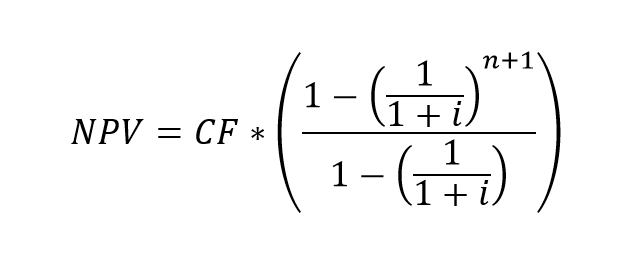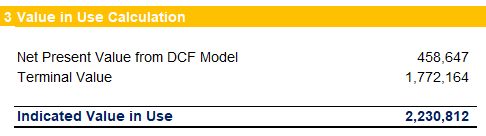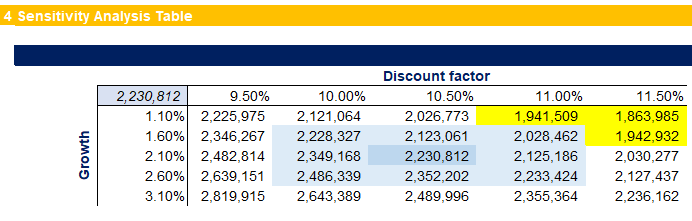## Using the Net Present Value (NPV) in Financial Analysis

The Net Present Value (NPV) is a profitability measure we use to figure out the present value of all expected future cash flows a project or investment will generate, including the initial capital we invest. It shows us the difference between the current value of cash inflows and outflows over a period.

## Net Present Value (NPV)

NPV, also known as Net Present Worth (NPW), is most prevalent in capital budgeting, where analysts use it to identify the projects with the highest value proposition for the business.

The Net Present Value is the method we use to get today’s value of a projected future cash flow stream. The measure is useful when comparing investment alternatives of similar nature. It relies on a discount rate, which represents the cost of capital to finance the endeavor. The main disadvantages of the NPV calculation lie with the fact that it makes assumptions for future events that may not occur.

## Calculating the NPV

We determine the Net Present Value of a series of cash flows by calculating the costs (negative cash flows) and benefits (positive cash flows). We achieve this via the following formula:Where:

• CFt is the cash flow in the period;
• n is the number of periods;
• t is the current period;
• i is the discount rate.

And whenever we have a constant cash flow each year, the formula can be represented as a standard finite geometric series:Where:

• CF is the continuous cash flow each period;
• n is the number of periods;
• i is the discount rate.

### Discount factor

We know that money now is worth more than the same amount in the future, also known as the Time Value of Money concept. To account for the decreasing value of money over time, we add a discount element in the NPV calculation.

We discount the cash flows to address the time value of money concept and to adjust for the inherent risk of the investment opportunity. We need to account for risk because different prospects have different risk levels. It is more likely to receive cash flows from government bonds than from a fintech startup. Therefore the discount rate includes a risk element and is usually higher for riskier investments.

There is a variety of rates we can use for the discount factor in the calculation above. One option is to use the expected return of other projects with similar risk or financing costs. We would typically use a company-specific discount factor that reflects the source of funds for the company. It might be the firm’s Weighted Average Cost of Capital (WACC), a set hurdle rate, or the interest rate, at which the business finances its other projects. Another option can be to use the interest rate the funds can generate if invested elsewhere. And in situations where capital is highly limited, we can use the reinvestment rate (the general rate at which the company invests), to reflect the opportunity cost of the investment.

Many analysts would also use a more significant discount rate to account for risk, opportunity costs, and other factors. It is essential to make sure we select our discount factor with the purpose of our analysis in mind.

### Periodic Rate

In some circumstances, the periods for our cash flow projections may differ from the standard one-year bucket. Whenever we face such a discrepancy between the available discount rate and the length of the periods in our calculation, we need to convert the annual rate to a periodic rate. We do so by employing the following formula:Where:

• r is the yearly discount rate;
• n is the number of periods per annum (weeks, months, quarters).

### NPV Functions in Excel

We can use two functions to calculate the Net Present Value in Microsoft Excel – NPV and XNPV.

NPV takes a stream of cash flows and a discount rate. XNPV is the more robust of the two, as it allows for varying time intervals between the expected cash flows, while NPV assumes the same regular interval.

## Net Present Value Analysis

The NPV analysis is a form of intrinsic valuation. We use it extensively across financial analysis and accounting to determine the value of a business, investment opportunities, capital projects, new ventures, and cost reduction plans.

We can apply the technique to pretty much anything that involves future cash flows. The calculation gives us the present discounted value of all future cash inflows less the cash outflow in the initial period (purchase price, implementation cost, initial investment amount), as well as any expected additional expenditures over the life of the endeavor.

A positive NPV is an indication that the current value of the future cash inflows exceeds the current value of the expected future cash outflows. Following this, we can assume that a positive Net Present Value would suggest a profitable project or investment. This is the basis of the ‘Net Present Value Rule,’ which states that investors should consider only ventures with a positive NPV.

A negative Net Present Value does not necessarily mean the project is generating losses. It may be contributing significantly towards accounting profit and net income. If the NPV is below zero, this means the project does not assist with value creation, as the rate of return is below the discount rate (or hurdle rate).

In real life, we mostly use the Net Present Value to compare capital projects within the same company. It is a popular tool for most financial analysts, as it considers the time value of money concept and provides a hard number that is easy to read and compare.

The Net Present Value is an indicator of how much value the investment or project will add to the company. There are three main options we can summarize as follows:### Net Present Value in Financial Modeling

There are two common ways we employ the NPV measure in our financial modeling efforts.

The first is to value a capital project, like building a new plant or implementing a new quality control system. To achieve this, we first forecast all relevant costs and income for the periods within the life span of the project. Then we can discount the net cash flows to their Net Present Value. We usually do so using the Weighted Average Cost of Capital of the company or a specific hurdle rate. By calculating the NPV, we can achieve two goals:

• Estimate if the project will create value for the business;
• Compare the venture to similar opportunities and select the most profitable one, when we lack resources to pursue multiple prospects at the same time.

The other scenario where we apply the NPV method is when we value a business. This requires us to build a detailed Discounted Cash Flows model with all costs and revenues, capital expenditures, working capital changes, and other detailed information. We start by setting the model assumptions, which is by far the most challenging part of the modeling process. Commonly, we will create a five-year forecast of the three statements (Income Statement, Balance Sheet and Cash Flow Statement). These will become the basis of a Free Cash Flow calculation, followed by a Terminal Value estimation, to cover the period after the five-year plan. In the end, we will discount the net cash flows at the company’s WACC or our investment portfolio hurdle rate to arrive at the estimated present value of the business.

## Internal Rate of Return (IRR) vs. NPV

The Internal Rate of Return (IRR) measure is similar to the Net Present Value calculation. IRR is the discount rate that reduces the NPV of an investment to zero. It is useful to compare projects with different lives or initial capital investment.

For example, an investment that pays out € 15 thousand per year over a period of 10 years, discounted at 10%, has a Net Present Value of around € 92 thousand. Reversed, this means € 92 thousand is a reasonable price for acquiring an investment that will achieve an IRR of 10%. Anything less than € 92 thousand will yield a higher return.

The IRR is the discount factor at which the NPV equals zero. It’s the profitability if the present value of the future cash inflows equals the current cost of the investment.

While very powerful, the NPV calculation relies heavily on some assumptions that may represent a substantial room for error:

• Cost of investment – it is easy to omit some of the initial costs, as we did not plan for some aspect of the project;
• Discount Factor – using a constant discount rate for the whole project, when in real life it’s hard to avoid changes;
• Projected returns – we might overstate the future cash flows because we are excited about the endeavor and thus overoptimistic;
• Potential unforeseen expenditures during the life span of the project.

When we calculate the Net Present Value of a cash flow stream we might run into some drawbacks. The measure is susceptible to small changes in the assumptions. Therefore, it is a good idea to perform a Sensitivity Analysis for our most important and impactful expectations. This high sensitivity also makes the  NPV metric easily manipulated to achieve the desired result. Performing additional checks of our assumptions is vital in preparing a robust NPV analysis. Still, we need to be careful, as it is easy to get lost in adding too many assumptions and sensitivity tables.

If the capital project includes high restoration costs in its end, these will be highly discounted, which can be an overly optimistic expectation. In such cases, we might want to explicitly include provisions for high losses in the last period of the project.

## Example NPV Calculation

This time we have a real-life model for you, so you can better understand how one might apply the NPV method in financial modeling and analysis.

I have anonymized and aggregated the data a bit, but other than that, it’s a valuation model I performed for a client recently.

We have the opportunity to acquire a business for € 1.95 B, that’s the asking price. To understand if the price is reasonable, we prepared a valuation model. First, we started by creating a five-year forecast and using the values to calculate the Free Cash Flow of the business for the next five years, as well as for the Terminal Value calculation.Using the NPV formula, we arrive at a Present Value of € 458 mil, by using the company’s WACC as a discount rate. We apply the expected growth rate of 2.10% to the Free Cash Flow in the final fifth period to calculate the basis for our Terminal Value calculation.The Indicated Value in Use, our valuation, arrives at € 2.23 B. It is way above the asking price, which suggests that acquiring the business is a sound investment decision.As we know the limitations and drawbacks of the NPV metric, we also add a sensitivity analysis calculation for our most important assumptions, the discount rate, and the growth percentage.The Indicated Value in Use in our analysis varies from € 2.82 B to € 1.86 B. It is essential to investigate further our assumptions for the discount factor and growth rate. As is evident from the highlighted values above, if our growth rate drops to 1.10% and our discount rate rises to more than 11.00% (or 1.60% growth rate and discount rate above 11.50%), the current value of the business will drop below its asking price.

This illustrates how important it is to perform sensitivity analysis and then further analyze our assumptions.

## Conclusion

The Net Present Value (NPV) is a robust analysis tool, as it considers all revenues, operating, and capital expenses from the project or investment. The measure also reflects the timing of cash flows, which can have a significant impact on the present value of an investment, because of the time value of money concept.

NPV is a valuable tool in our financial modeling and analysis toolkit. Still, we need to be aware of its drawbacks and always use it in corroboration with other metrics and valuation methods.

## Get a FREE Excel Benchmark Analysis Template## FCCA, FMVA

Hi! I am a finance professional with 10+ years of experience in audit, controlling, reporting, financial analysis and modeling. I am excited to delve deep into specifics of various industries, where I can identify the best solutions for clients I work with.

In my spare time, I am into skiing, hiking and running. I am also active on Instagram and YouTube, where I try different ways to express my creative side.

The information and views set out in this publication are those of the author(s) and do not necessarily reflect the official opinion of Magnimetrics. Neither Magnimetrics nor any person acting on their behalf may be held responsible for the use which may be made of the information contained herein. The information in this article is for educational purposes only and should not be treated as professional advice. Magnimetrics and the author of this publication accept no responsibility for any damages or losses sustained in the result of using the information presented in the publication.